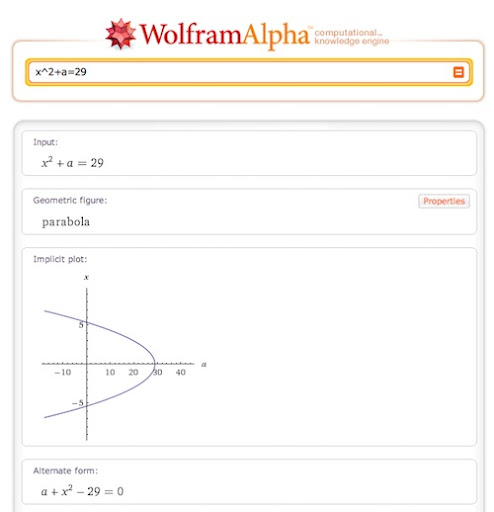### Updated: Wolfram Alpha can help parents with children's math homework -- also Khan Academy

Updated (3/2/2011 @ 250pm): I have added a link to an algebra video from Khan Academy to the end of this post. One of my Facebook friends recommended it and this video is EXACTLY the type of equations that my son is studying right now. I plan on introducing him to several of these videos. They are very clear and I think they really help to develop a deeper understanding of the material.

My son is about to turn 13 and his current math lessons include the beginnings of algebra. To say the least, my knowledge of algebra is almost non-existent. I haven't even thought about it much since I left high school, where I barely made it through the required classes. Of course, as a responsible parent, someone needs to assist with math homework and that task has fallen to me, even with my weak skills.

After struggling through a series of homework pages where I was learning as much as him, I really needed a way to check our work. While the book provides some answers, the teacher, for good reason, usually selects those problems that are not answered. How was I to know that we had come up with the right solution?

Enter WolframAlpha. WolframAlpha garnered a lot of attention at its launch due to the unique approach it took to searching the Internet. Instead of returning results as a series of web pages the reader could link to, it tries to provide answers directly on the search page. One specialized feature is that Wolfram Alpha will also solve equations. I can type in x^2+a=29 and it will solve it for me, even showing me geometrical representation, when appropriate. It understands "order of operations" and many complex mathematical concepts much better than I ever will.The site also helps me with one of my personal math failings -- dealing with negative numbers. I can put in a string of calculations from the math worksheet and be reasonably sure that I haven't botched the typical "negative times a negative makes a positive", etc calculations that quickly lead to a very wrong answer.

You can check under the Examples tab on the web site if you are having trouble figuring out how to enter a particular equation or problem. Mathematical notation on computer is, by necessity, a bit different. Exponents are indicated using the ^ character and square roots by sqrt i.e. sqrt2. (See more)

WolframAlpha won't answer everything, of course, but it helps to reduce the number of questions I have to refer back to the teacher for further explanation. It also helps to tell if we are on the right track for a solution or running off into the weeds. The next time you are feeling a bit out of your element with math homework, give WolframAlpha a try.

I would love to hear about your experiences. Leave me a comment and tell me if it helps you as much as it has helped me.

Here is an algebra video from Khan Academy which is another great resource for helping your children with subjects that might be a little beyond your skill level.The only problem is any parabola is x^2 for is always vertical, not horizontal. For example. if a=3, then the plot would look like this: http://www.wolframalpha.com/input/?i=x^2%2B3%3D29
This is one of those cases where the results were misinterpreted because of the variable a. If you use x and y or just x, your input should be interpreted correctly.

Try these other inputs to see more:
x^2+y=29
x^2+x=-7
x^2=3-2xThanks for that. I would have never known. Of course, I made up the equation off the top of my head, so I will take the blame for the problem. (LAUGH)